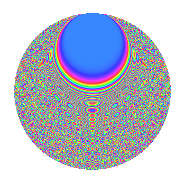# Properties

 Label 3024.2.exLevel 3024 Weight 2 Character orbit ex Rep. character $$\chi_{3024}(367,\cdot)$$ Character field $$\Q(\zeta_{18})$$ Dimension 864 Sturm bound 1152

# Related objects

## Defining parameters

 Level: $$N$$ = $$3024 = 2^{4} \cdot 3^{3} \cdot 7$$ Weight: $$k$$ = $$2$$ Character orbit: $$[\chi]$$ = 3024.ex (of order $$18$$ and degree $$6$$) Character conductor: $$\operatorname{cond}(\chi)$$ = $$756$$ Character field: $$\Q(\zeta_{18})$$ Sturm bound: $$1152$$

## Dimensions

The following table gives the dimensions of various subspaces of $$M_{2}(3024, [\chi])$$.

Total New Old
Modular forms 3528 864 2664
Cusp forms 3384 864 2520
Eisenstein series 144 0 144

## Trace form

 $$864q + O(q^{10})$$ $$864q + 36q^{29} - 72q^{65} - 216q^{69} + 72q^{77} + 72q^{81} + O(q^{100})$$

## Decomposition of $$S_{2}^{\mathrm{new}}(3024, [\chi])$$ into newform subspaces

The newforms in this space have not yet been added to the LMFDB.

## Decomposition of $$S_{2}^{\mathrm{old}}(3024, [\chi])$$ into lower level spaces

$$S_{2}^{\mathrm{old}}(3024, [\chi]) \cong$$ $$S_{2}^{\mathrm{new}}(756, [\chi])$$$$^{\oplus 3}$$

## Hecke Characteristic Polynomials

There are no characteristic polynomials of Hecke operators in the database